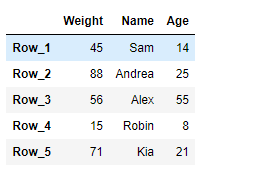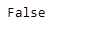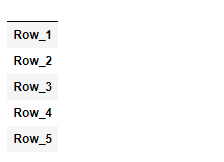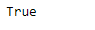# Python | Pandas DataFrame.empty

• Last Updated : 20 Feb, 2019

Pandas DataFrame is a two-dimensional size-mutable, potentially heterogeneous tabular data structure with labeled axes (rows and columns). Arithmetic operations align on both row and column labels. It can be thought of as a dict-like container for Series objects. This is the primary data structure of the Pandas.

Pandas` DataFrame.empty` attribute checks if the dataframe is empty or not. It return `True` if the dataframe is empty else it return `False`.

Attention geek! Strengthen your foundations with the Python Programming Foundation Course and learn the basics.

To begin with, your interview preparations Enhance your Data Structures concepts with the Python DS Course. And to begin with your Machine Learning Journey, join the Machine Learning - Basic Level Course

Syntax: DataFrame.empty

Parameter : None

Returns : bool

Example #1: Use `DataFrame.empty` attribute to check if the given dataframe is empty or not.

 `# importing pandas as pd``import` `pandas as pd`` ` `# Creating the DataFrame``df ``=` `pd.DataFrame({``'Weight'``:[``45``, ``88``, ``56``, ``15``, ``71``],``                   ``'Name'``:[``'Sam'``, ``'Andrea'``, ``'Alex'``, ``'Robin'``, ``'Kia'``],``                   ``'Age'``:[``14``, ``25``, ``55``, ``8``, ``21``]})`` ` `# Create the index``index_ ``=` `[``'Row_1'``, ``'Row_2'``, ``'Row_3'``, ``'Row_4'``, ``'Row_5'``]`` ` `# Set the index``df.index ``=` `index_`` ` `# Print the DataFrame``print``(df)`

Output :Now we will use `DataFrame.empty` attribute to check if the given dataframe is empty or not.

 `# check if there is any element``# in the given dataframe or not``result ``=` `df.empty`` ` `# Print the result``print``(result)`

Output :As we can see in the output, the `DataFrame.empty` attribute has returned `False` indicating that the given dataframe is not empty.

Example #2: Use `DataFrame.empty` attribute to check if the given dataframe is empty or not.

 `# importing pandas as pd``import` `pandas as pd`` ` `# Creating an empty DataFrame``df ``=` `pd.DataFrame(index ``=` `[``'Row_1'``, ``'Row_2'``, ``'Row_3'``, ``'Row_4'``, ``'Row_5'``])`` ` `# Print the DataFrame``print``(df)`

Output :Now we will use `DataFrame.empty` attribute to check if the given dataframe is empty or not.

 `# check if there is any element``# in the given dataframe or not``result ``=` `df.empty`` ` `# Print the result``print``(result)`

Output :As we can see in the output, the `DataFrame.empty` attribute has returned `True` indicating that the given dataframe is empty.

My Personal Notes arrow_drop_up